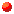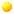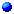# Factoring Tutorial```
In factoring any polymonial we take the following two step approach.
1. Look for common factor. 2. Classify by number of terms.
/ Difference of SquaresBinomials Sum of Cubes                   \ Difference of Cubes
/ Perfect SquareTrinomials                   \ Nonperfect SquarePolynomials of four or more terms - Generally handled in a more advanced course. ```